JalFaizy Shaikh — Published On June 27, 2017 and Last Modified On July 26th, 2020

## Introduction

It is one thing to learn data science by reading or watching a video / MOOC and other to apply it on problems. You need to do both the things to learn the subject effectively. Today’s article is meant to help you apply deep learning on an interesting problem.

If you are questioning, why learn or apply deep learning – you have most likely come out of a cave just now. Deep learning in already powering face detection in cameras, voice recognition on mobile devices to deep learning cars. Today, we will solve age detection problem using deep learning.

If you are new to deep learning, I would recommend you to refer the articles below before going through this tutorial and making a submission.

• Why Participate in a Practice Problem (Deep Learning)?
• What is the problem again?
• Let’s solve the problem!
• Intermission: Our first submission
• Let’s solve the problem! Part 2: Building better models
• Intermission: Visual Inspection of our predictions
• What’s Next?

## Why Participate in a Practice Problem (Deep Learning)?

If you have learnt or read about Deep learning in last few days / months, and are looking for applying your new skill – practice problems are the right place to start. I say so because they provide you an experience of solving problems from scratch without making them too competitive.

Here are the reasons why you should pick up a few practice problems:

• Have time to build the basics: I always suggest, we should always start with establishing a right foundation (Thinking about the problem statement and exploring the data set) and practice this a lot. I still see people start coding without thinking about the problem and understanding the data. In this approach, you actually don’t explore the problem and data because you are focusing on exploiting the algorithm directly.
• Peer Learning (Forum/ Blog): In practice problem, participants share their approach on forum or via blog and are always ready to discuss new approaches. People don’t hold back as this is not a prized competition.
• Practice: These practice problems are like your practice sessions before you go out and perform on real life problems. You should evaluate your performance here first and try to do well. Your main objective during this time should be to get the most out of the tool, algorithms and the dataset.
• Test your knowledge: It is a great place to try out something you learnt and it will be very beneficial to you. The result don’t matter as much as this is a practice problem.

## What is the problem again?

The first step to do when participating in a hackathon is to understand the problem. In this article, we will look at a recently published practice problem:  Age Detection of Indian Actors. You can view the problem statement on the hackathon page, but I will briefly mention it here.

The task is to predict the age of a person from his or her facial attributes. For simplicity, the problem has been converted to a multi-class problem with classes as Young, Middle and Old.

Seems easy at a first glance right?

If you actually see the data, it seems hard even for a human! Lets check for ourselves! Here are some random good examples from our data.

Middle Aged: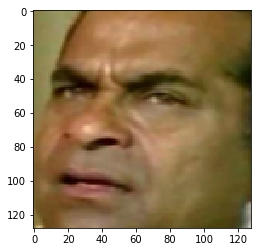Old: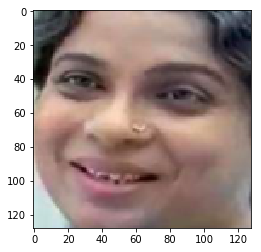Young: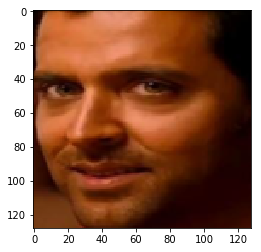But can you guess these?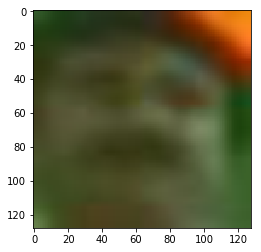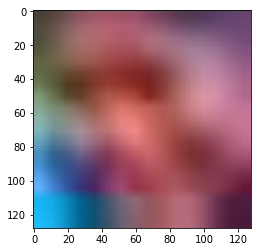Apparently both are middle aged actors!

To solve these problems, you need to have a streamlined approach. We will see this in the next section.

## Let’s solve the problem!

Now that you know the problem, let us get started. I assume you have numpy, scipy, pandas, scikit-learn and keras installed. If you don’t, please install them. The articles above should help you.

First things first; let us download the data and load it into our jupyter notebooks! If you haven’t already been introduced; here is the link to the practice problem (https://datahack.analyticsvidhya.com/contest/practice-problem-age-detection/).

Before building a model, I urge you to solve this simple exercise:

Can you write a script that will randomly load an image into jupyter notebook and print it? (PS: Don’t look at the answer below!). Post your code in this discuss thread.

Here’s my approach to the exercise; As always, I first imported all the necessary modules,

```% pylab inline
import os
import random

import pandas as pd

Then I loaded the csv files, so that it would be easier to locate the files

```root_dir = os.path.abspath('.')
data_dir = '/mnt/hdd/datasets/misc'

Then I wrote a script to randomly choose an image and printed it

```i = random.choice(train.index)

img_name = train.ID[i]

print(‘Age: ‘, train.Class[i])```
`imshow(img)`

Here’s what I got:

Age:  YOUNG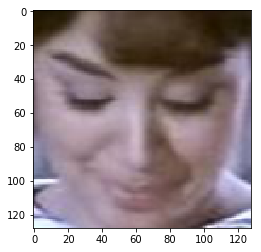The motive of this exercise was that you can randomly view the dataset and check what problems you could possibly face when building the model.

Here are a few of my hypotheses of what problems we could face

• Variations in shape: One image had a shape (66, 46) whereas other had (102, 87)
• Multiple viewpoints: We have faces with whichever view possible! Here are some examples:

side view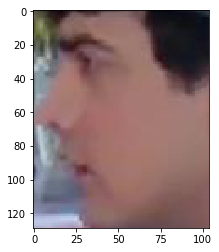front view• Quality of images: Some images were found to be too pixelated. Here’s an example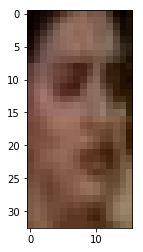• Discrepancy in brightness and contrast: Check the images below; do they seem to make your problem easier to solve?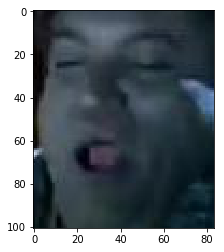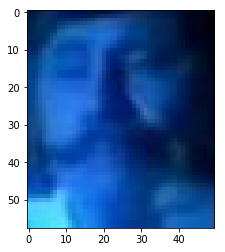For now, let us focus on only one problem, viz how to handle variations in shape?

We can do this by simply resizing the image. Let us load all the images and resize them into a single numpy array

```from scipy.misc import imresize

temp = []
for img_name in train.ID:
img_path = os.path.join(data_dir, 'Train', img_name)
img = imresize(img, (32, 32))
img = img.astype('float32') # this will help us in later stage
temp.append(img)

train_x = np.stack(temp)
```

And similarly for test images

```temp = []
for img_name in test.ID:
img_path = os.path.join(data_dir, 'Test', img_name)
img = imresize(img, (32, 32))
temp.append(img.astype('float32'))

test_x = np.stack(temp)```

We can do one more thing that could help us build a better model; i.e. we can normalize our images. Normalizing the images will make our train faster.

```train_x = train_x / 255.
test_x = test_x / 255.```

Now let’s take a look at our target variable. I have another exercise for you; What is the distribution of classes in our data? Could you say it is a highly imbalanced problem?

Here’s my try for the exercise;

```train.Class.value_counts(normalize=True)

MIDDLE    0.542751
YOUNG     0.336883
OLD       0.120366
Name: Class, dtype: float64```

## Intermission: Our first submission!

On the basis of distribution of our data, we can create a simple submission. We see that most of the actors are middle aged. So we can say that all the actors in our test dataset are middle aged!

```test['Class'] = 'MIDDLE'
test.to_csv(‘sub01.csv’, index=False)```

Upload this file on the submission page to see the result!

## Let’s solve the problem! Part 2: Building better models

Before creating something substantial, let us bring our target variable in shape. We will convert our target into dummy columns so that it will be easier for our model to ingest it. Here’s how I would do it.

```import keras
from sklearn.preprocessing import LabelEncoder

lb = LabelEncoder()
train_y = lb.fit_transform(train.Class)
train_y = keras.utils.np_utils.to_categorical(train_y)```

Now comes the main part, building a model! As the problem is related to image processing, it is wiser to use neural networks to solve the problem. We will too build a simple feedforward neural network for this problem.

First we should specify all the parameters we will be using in our network

```input_num_units = (32, 32, 3)
hidden_num_units = 500
output_num_units = 3

epochs = 5
batch_size = 128```

Then we will import the necessary keras modules

```from keras.models import Sequential
from keras.layers import Dense, Flatten, InputLayer```

After that, we will define our network

```model = Sequential([
InputLayer(input_shape=input_num_units),
Flatten(),
Dense(units=hidden_num_units, activation='relu'),
Dense(units=output_num_units, activation='softmax'),
])```

To see how our model looks like; lets print it

`model.summary()`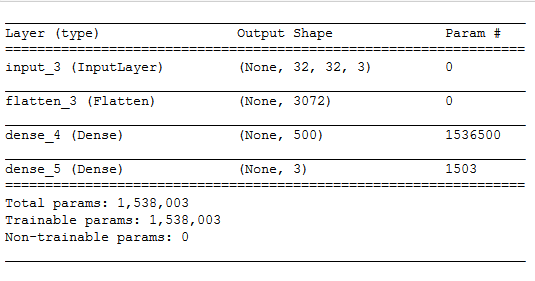Now lets compile our network and let it train for a while

```model.compile(optimizer='sgd', loss='categorical_crossentropy', metrics=['accuracy'])
model.fit(train_x, train_y, batch_size=batch_size,epochs=epochs,verbose=1)```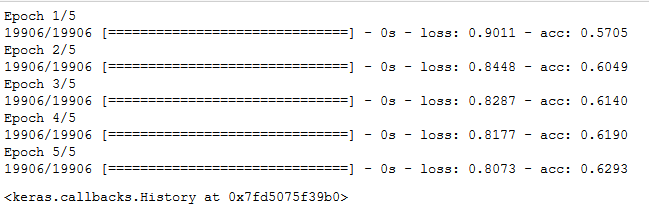Seems like its training! But we still haven’t validated it. Validation is necessary if we want to ensure that our model will perform well on both the data it is training on and on a new testing data

Let’s tweak the code a little bit to cross validate it.

`model.fit(train_x, train_y, batch_size=batch_size,epochs=epochs,verbose=1, validation_split=0.2)`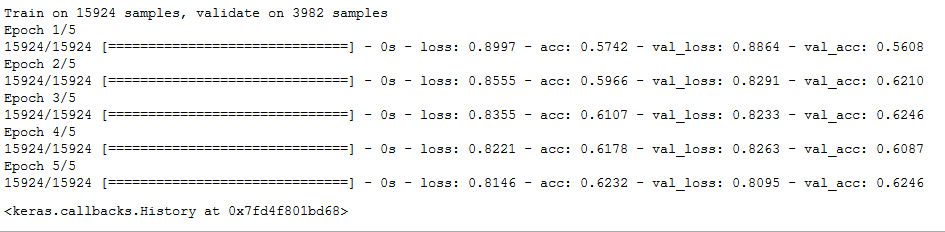The model seems to perform good for a first model. Lets submit the result.

```pred = model.predict_classes(test_x)
pred = lb.inverse_transform(pred)

test['Class'] = pred
test.to_csv(‘sub02.csv’, index=False)
```

## Intermission: Visual Inspection of our predictions

Here’s another simple exercise for you; Print the image along with the predictions of your trained model. Do this preferably on your training dataset so that you can check your predictions along with the real target

Here’s my take on the exercise,

```i = random.choice(train.index)
img_name = train.ID[i]

imshow(imresize(img, (128, 128)))
pred = model.predict_classes(train_x)
print('Original:', train.Class[i], 'Predicted:', lb.inverse_transform(pred[i]))

Original: MIDDLE Predicted: MIDDLE```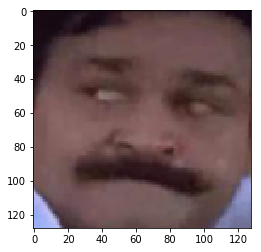## What’s Next

We have built a benchmark solution with a simple model at hand. What more can we do?

Here are some tips I can suggest

• A better neural network model can give you a great boost. You can try using a convolutional neural network which is better suited for image related problems. Here’s a simple CNN for your reference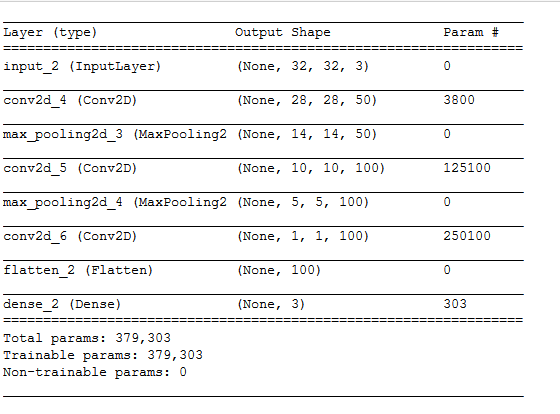• We have not let the model train much. You can increase the number of epochs our model trains for. There are multiple hyperparameters in a neural network you can tune. Here’s a guide that will help you to tune them (https://www.analyticsvidhya.com/blog/2016/10/tutorial-optimizing-neural-networks-using-keras-with-image-recognition-case-study/)
• We mentioned a few problems we could face while solving the problem. Most of them would be minimized if we do proper preprocessing of the data.
• One thing you could try is converting color images to grayscale. This is because color is not that important a feature when you are solving the problem. More of these intuitions might come to you when you understand the problem better.

## End Notes:

In this article, I have explained a simple benchmark solution for Age Detection Practice Problem. There’s many things you could do which even I haven’t mentioned in the article. You can suggest them in the comments below!###### JalFaizy Shaikh

Faizan is a Data Science enthusiast and a Deep learning rookie. A recent Comp. Sc. undergrad, he aims to utilize his skills to push the boundaries of AI research.

## 25 thoughts on "Hands on with Deep Learning – Solution for Age Detection Practice Problem"###### Rajneesh Tiwari says:June 27, 2017 at 10:35 am
Would love to see the winner's approach in this data hack. @Kunal - Would it be possible for you to share once the competition is closed. Reply###### vijay says:June 27, 2017 at 12:11 pm
Very Superb Article. Thanks a lot for sharing this information Reply###### Faizan Shaikh says:June 27, 2017 at 12:51 pm###### Faizan Shaikh says:June 27, 2017 at 12:53 pm
Hi Rajneesh, Age detection is a practice problem, so everyone is free to share their approach to the problem. Reply###### Ravi Theja says:June 27, 2017 at 1:21 pm
Much needed article. Thanks for sharing. Reply###### Sayak Paul says:June 27, 2017 at 4:53 pm
Excellent article. You kinda saved me. Reply###### Faizan Shaikh says:June 27, 2017 at 11:11 pm###### Faizan Shaikh says:June 27, 2017 at 11:12 pm###### Ram says:June 28, 2017 at 12:02 am###### Afzal says:June 28, 2017 at 11:24 am
Thanks for your article. All the article related to some applications are simply writing the keras functions like Flatten(), to_categorical (), label Encoder() etc. with out giving any idea about "why using that functions" . It will be better if you include those details in your next articles. Thank you Reply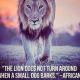###### olufemi says:July 03, 2017 at 7:43 pm
Great tutorial..... How can I do this in R? Haven't seen any Keras deep learning tutorials in R on your Site....and not that many elsewhere either. Reply###### P Mitra says:July 04, 2017 at 12:07 pm
Hi, I have run the first example code in Ubuntu Linux. It ran successfully but it only prints the ('Age:', 'YOUNG') part. It does not show the image as you have mentioned above. Please can you suggest ? Reply###### Faizan Shaikh says:July 08, 2017 at 6:02 pm
Hi P Mitra, The sequence of the two lines was incorrect. I have updated the article with your feedback. Reply###### Faizan Shaikh says:July 08, 2017 at 6:04 pm###### Faizan Shaikh says:July 08, 2017 at 6:06 pm
Thanks for the feedback Afzal. Actually I deliberately miss out most of this because it is already covered in articles which I have given for pre-reference (eg. https://www.analyticsvidhya.com/blog/2016/10/tutorial-optimizing-neural-networks-using-keras-with-image-recognition-case-study/) Reply###### Vivek says:July 14, 2017 at 5:11 pm
Hi Faizan, Thanks for your article, From it, we can get initial idea to approach deep learning problem. Further I wanted to know what are the possible ways in which model can predict the real image age detection. Thanks for your knowledge shared:) Reply###### goxul says:August 15, 2017 at 11:32 am
Hey Faizan, Is there a way to download this dataset on cloud? I use AWS to train my models and I have been unable to download the data on my server. Reply###### Faizan Shaikh says:August 17, 2017 at 4:54 pm###### Faizan Shaikh says:August 17, 2017 at 4:59 pm
You are welcome! Regarding your query, could you clarify re you referring to deep learning solutions or solving age detection in general? Reply###### Gokul says:August 17, 2017 at 6:48 pm###### Manasa says:August 27, 2017 at 12:10 pm
Hi Faizan, Thanks for the article. I am eager to solve this problem however I was wondering if one would need a GPU to train 35K images. I currently don't have GPU support. Please let me know. Thanks. Reply###### Faizan Shaikh says:August 28, 2017 at 7:54 pm
You dont necessarily need a GPU to participate. Its just that when running the models on CPU, you would take much more time train Reply###### Rajneesh Tiwari says:September 11, 2017 at 11:36 am
You can do batch processing, by not loading the entire data-set at once but rather feeding your algorithm a set of batches (say 128 images). This will take more time, but will consume less memory (CPU or GPU). Reply###### Sebastian says:April 17, 2018 at 12:24 pm
Can we do Age detection problem in r? Reply###### Aishwarya Singh says:April 19, 2018 at 2:07 pm
Hi Sebastian, Yes you can solve this problem in R as well. Reply